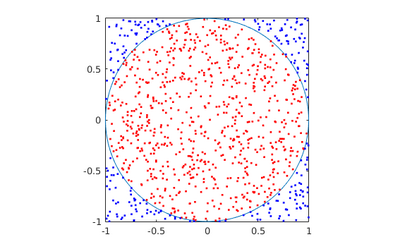# Course:CPSC522/MCMC

Jump to navigation Jump to search

## Markov Chain Monte Carlo (MCMC)

MCMC is a class of Markov chain-based algorithms used to generate samples for use in Monte Carlo integration.

Principal Author: Ricky Chen
Collaborators: Jiahong Chen, Yan Zhao

## Abstract

Monte Carlo Integration is briefly reviewed, and Markov Chain Monte Carlo is introduced as a method for solving Monte Carlo Integration when the samples are in high dimensions. We briefly review Monte Carlo Integration. Then we describe the theoretical justifications behind MCMC algorithms, and outline the generic Metropolis-Hastings algorithm and the commonly used Gibbs sampler.

### Background

Knowledge of probability distributions, expectations, and basic properties of Markov chains are assumed.

## Monte Carlo Integration

The goal of Monte Carlo integration is to approximate $E[f(X)]$where $X$is a possibly-multidimensional random variable from some target distribution $\pi$. Due to the definition of expectation, this means we can approximate any integral of the form

$E[f(X)]=\int f(x)\pi (x)dx$Where $\pi$satisfies the properties of a density function.

### ExampleApplied Monte Carlo integration on estimating the area of a unit circle. The red samples are within the circle and the blue samples are outside. The fraction of red samples is the ratio $\pi /4$Suppose we want to find the area within the unit circle and denote this area by $K$. This can be written as an integral over the space $\Omega =[-1,1]\times [-1,1]$.

$K=\int _{\Omega }\mathbf {1} _{x^{2}+y^{2}\leq 1}d(x,y)$Where $\mathbf {1} _{A}$is the indicator function

$\mathbf {1} _{A}:={\begin{cases}1&{\text{if }}A{\text{ is true}}\\0&{\text{if }}A{\text{ if false}}\end{cases}}$First we must transform this integral to the form that Monte Carlo integration can solve by introducing a random variable $(X,Y)$that is uniform over the space $\Omega$. Notice that the area of $\Omega$is

$\int _{\Omega }d(x,y)=4$Thus the uniform variable $(X,Y)$over $\Omega$has density function $\pi (x,y)=1/4$. We can now write

$E[f(X,Y)]=\int _{\Omega }f(x,y)\pi (x,y)d(x,y)=(1/4)\int _{\Omega }f(x,y)d(x,y)=K/4$Where we define $f(x,y):=\mathbf {1} _{x^{2}+x^{2}\leq 1}$.

The simplest Monte Carlo simulation involves sampling $N$times from the distribution of $\pi$, computing the value of $f$for each sample, and then averaging to approximate $E[f(X,Y)]$.

${\frac {1}{N}}\sum _{i=1}^{N}f(X_{i},Y_{i})\approx E[f(X,Y)]$This Monte Carlo simulation written as a Matlab function:

   function [estimate] = simPi(N)
f = @(x,y) x^2 + y^2 <= 1;
sum = 0;
for i=1:N
x = rand*2-1;
y = rand*2-1;
sum = sum + f(x,y);
end
estimate = 4*sum/N;


With a high enough $N$, we get a decent estimate of $K\approx \pi$(the constant 3.1415...).

## Monte Carlo Integration Based on Markov Chains

The example above only samples from uniform distributions. However, when the distribution of interest is hard to sample from using basic Monte Carlo methods, MCMC is useful if we don't know how to explicitly sample from the target distribution $\pi$. At its core, MCMC methods make use of the following property of Markov chains.

Law of Large Numbers for Markov Chains
Let $X_{n}$be a Markov chain in ${\mathcal {X}}$.
Suppose $X_{n}$is homogeneous, irreducible, and the stationary distribution exists, then for any function $f:{\mathcal {X}}\rightarrow \mathbb {R}$,
${\frac {1}{N}}\sum _{i=1}^{N}f(X_{n})\rightarrow E[f(X_{\infty })]$Where $X_{\infty }\sim \pi$is the stationary distribution of the Markov chain.

Thus we can perform Monte Carlo integration by designing a Markov chain such that the stationary distribution exists and is the target distribution $\pi$, then we can simulate the Markov chain to obtain "samples". The law of large numbers assures us that the estimator ${\frac {1}{N}}\sum _{i=1}^{N}f(X_{n})$is consistent, ie reaches the exact value as $N$goes to infinity.

## Metropolis-Hastings (MH) AlgorithmIllustration of a MCMC method sampling from the target distribution (grey). We wish to obtain samples (blue) from this distribution. The green path indicates the transitions of the MCMC method. While the method starts off on the tail of the target distribution, it eventually gets to the area of high densities and randomly traverses the space.

We begin by describing the most general algorithm, which does not make any assumptions for $\pi$, other than that we can evaluate it pointwise. The Metropolis-Hastings algorithm often acts as a base for numerous other MCMC methods.

MH inputs:

1. A target distribution $\pi :{\mathcal {X}}\rightarrow \mathbb {R}$that we can evaluate pointwise.
2. A proposal distribution/transition $q(x\rightarrow y)$from which we can simulate $q(x\rightarrow \cdot ):{\mathcal {X}}\rightarrow \mathbb {R}$and evaluate pointwise.

MH algorithm:

1. Initialize $x^{0}$arbitrarily and set $F\leftarrow 0$2. Loop $i=1,2,3,\dots ,N$(until enough samples are generated):
(a) Propose a new state, $x'\sim q(x^{(i=1)}\rightarrow \cdot )$(b) Compute
$A(x^{(i-1)}\rightarrow x')=\min \left\{1,{\frac {\pi (x')q(x'\rightarrow x^{(i-1)})}{\pi (x^{(i-1)})q(x^{(i-1)}\rightarrow x'))}}\right\}$(c) Accept $x'$as the new state $x^{(i)}$with probability $A(x^{(i-1)}\rightarrow x')$; otherwise, accept $x^{(i-1)}$as the new state.
(d) $F\leftarrow F+f(x^{(i)})$3. Return $F/N$The MH algorithm traverses the space of ${\mathcal {X}}$where the step direction is proposed by $q(y|x)$but the decision of whether to take the step is decided based on the acceptance ratio $A(x^{(i-1)}\rightarrow x')$. The acceptance ratio increases if the proposed state $x'$is in a region with high density, and decreases if the proposed state is in a region of low density based. This makes sense intuitively since we would want more samples from a high density region.

#### Proof of Correctness

To prove that the MH algorithm does indeed simulate a Markov chain with stationary distribution $\pi$, we prove a stronger condition, the detailed balance equation which says for any $x,y\in {\mathcal {X}}$,

$\pi (x)p(x\rightarrow y)=\pi (y)p(y\rightarrow x)$Where $p(x\rightarrow y)$is the probability of transitioning from $x$to $y$. The detailed balance equation implies that $\pi$is the stationary distribution. To see this, simply take the sum over $x$on both sides.

$\sum _{x}\pi (x)p(x\rightarrow y)=\sum (x)\pi (y)p(y\rightarrow x)\implies \sum _{x}\pi (x)p(x\rightarrow y)=\pi (y)$Detailed balance is trivially true for $x=y$. First see that for $x\neq y$, the transition function is the probability of sampling $y$at step 2(a) times the probability of acceptance

$p(x\rightarrow y)=q(y|x)A(x\rightarrow y)$The proof of detailed balance for the MH algorithm is very simple:

$\pi (x)p(x\rightarrow y)=\pi (x)q(y|x)A(x\rightarrow y)$$\pi (x)p(x\rightarrow y)=\min\{\pi (x)q(y|x),\pi (y)q(x|y)\}$$\pi (x)p(x\rightarrow y)=\min\{\pi (y)q(x|y),\pi (x)q(y|x)\}$$\pi (x)p(x\rightarrow y)=\pi (y)q(x|y)A(y\rightarrow x)$$\pi (x)p(x\rightarrow y)=\pi (y)p(y\rightarrow x)$Thus applying law of large numbers, we've proven the correctness of the MH algorithm. Similar proofs are constructed for other MCMC algorithms.